## Pictures of Primes II

### Primes 10^50 < p < 10^50 + 2^15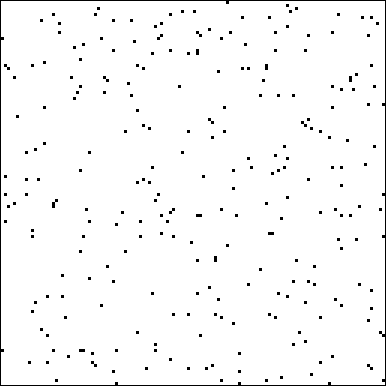In the above 128 x 128 matrix the n-th square is black iff the number 10^50 + 2n + 1 is prime. By the prime number theorem, the density of primes in this range is approximatively 1/log(10^50) = 1/115, so we would expect about 2^15/115 = 285 primes in this picture. The actual number is 269. The first prime after 10^50 is 10^50 + 151.
There are three twin primes (10^50 + x_i, 10^50 + x_i + 2) in this picture, with x_i = 18307, 19891, 29749. This is in good agreement with a conjecture of Hardy and Littlewood, which gives the density of twin primes as 1.32/log(x)^2. The expected number of twin primes in our interval of length 2^15 = 32768 according to this conjecture is 3.26.. .

### Primes 10^100 < p < 10^100 + 2^15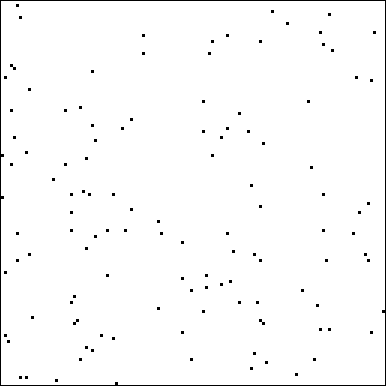### Primes 10^200 < p < 10^200 + 2^15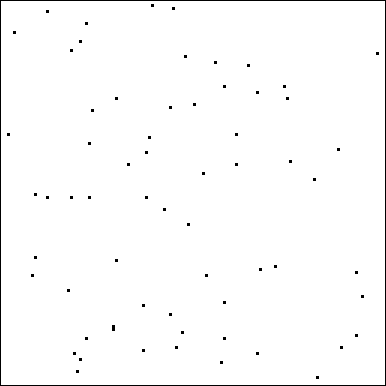### Primes 10^400 < p < 10^400 + 2^15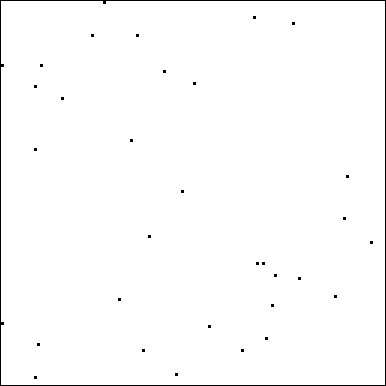` smaller primes `
Otto Forster (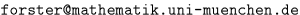), 95-05-29/97-04-30